# Band Pass Filter: Circuit & Transfer Function (Active & Passive)

Contents

## What is a Band Pass Filter?

A band pass filter (also known as a BPF or pass band filter) is defined as a device that allows frequencies within a specific frequency range and rejects (attenuates) frequencies outside that range.

The low pass filter is used to isolate the signals which have frequencies higher than the cutoff frequency. Similarly, the high pass filter is used to isolate the signals which have frequencies lower than the cutoff frequency.

By the cascade connection of high pass and low pass filter makes another filter, which allows the signal with specific frequency range or band and attenuate the signals which frequencies are outside of this band. This type of filter is known as Band Pass Filter.

The Band Pass Filter has two cutoff frequencies. The first cutoff frequency is from a high pass filter. This will decide the higher frequency limit of a band that is known as the higher cutoff frequency (fc-high). The second cutoff frequency is from the low pass filter. This will decide the lower frequency limit of the band and that is known as lower cutoff frequency (fc-low).

## Band Pass Filter Circuit

The band pass filter is a combination of low pass and high pass filters. Therefore, the circuit diagram contains the circuit of high pass and low pass filters. The circuit diagram of the passive RC band pass filter is as shown in the below figure.

The first half of the circuit diagram is a passive RC high pass filter. This filter will allow the signals which have frequencies higher than the lower cutoff frequency (fc-low). And attenuate the signals which have frequencies lower than (fc-low).The second half of the circuit diagram is a passive RC low pass filter. This filter will allow the signals which have frequencies lower than the higher cutoff frequency (fc-high). And it will attenuate the signals which have frequencies higher than (fc-high).The band or region of frequency in which the band pass filter allows the signal to pass that is known as Bandwidth. The bandwidth is a difference between the higher and lower value of cutoff frequency.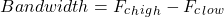## Band Pass Filter Types

There are many types of band pass filter circuits are designed. Let’s explain the major types of filter circuits in detail.

### Active Band Pass Filter

The active band pass filter is a cascading connection of high pass and low pass filter with the amplifying component as shown in the below figure.

The circuit diagram of Active Band Pass Filter is divided into three parts. The first part is for a high pass filter. Then the op-amp is used for the amplification. The last part of the circuit is the low pass filter. The below figure shows the circuit diagram of Active Band Pass Filter.

### Passive Band Pass Filter

The passive filter used only passive components like resistors, capacitors, and inductors. Therefore, the passive band pass filter is also used passive components and it does not use the op-amp for amplification. So, like an active band pass filter, the amplification part is not present in a passive band pass filter.

The passive band pass filter is a combination of passive high pass and passive low pass filters. Hence, the circuit diagram also contains circuits of high pass and low pass filters.

The first half of the circuit is for the passive high pass filter. And the second half is for the passive low pass filter.

### RLC Band Pass Filter

As the name suggests RLC, this band pass filter contains only resistor, inductor and capacitor. This is also a passive band pass filter.

According to the connection of RLC, there are two circuit configurations of the RLC band pass filter. In the first configuration, the series LC circuit is connected in series with the load resistor. And the second configuration is parallel LC circuit is connected in parallel with a load resistor.

The bandwidth for the series and parallel RLC band pass filter is as shown in the below equations.

Bandwidth for series RLC filter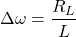The equation of corner frequency is the same for both configurations and the equation is

Bandwidth for parallel RLC filter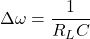### Wide Band Pass Filter

According to the size of bandwidth, it can divide into a wide bandpass filter and a narrow bandpass filter. If the Q-factor is less than 10, the filter is known as a wide pass filter. As the name suggests, the bandwidth is wide for the wide bandpass filter.

In this type of filter, the high pass and low pass filter are different sections as we have seen in the passive band pass filter. Here, both filters are passive.

Another circuit arrangement can be done by using an active high pass and an active low pass filter. The circuit diagram of this filter is as shown in the below figure where the first half is for active high pass filter and the second half is for active low pass filter.

Because of the different parts of filters, it is easy to design the circuit for a wide range of bandwidth.

### Narrow Band Pass Filter

The band pass filter which has a quality factor greater than ten. The bandwidth of this filter is narrow. Therefore, it allows the signal with a small range of frequencies. It has multiple feedback. This band pass filter uses only one op-amp.

This band pass filter is also known as multiple feedback filter because there are two feedback paths.

In this band pass filter, the op-amp is used in non-inverting mode. The circuit diagram of band pass filter is as shown in the below figure.

Below figure differentiate the frequency response between wide pass and narrow pass filter.

## Band Pass Filter Transfer Function

### First Order Band Pass Filter Transfer Function

A first order band pass filter is not possible, because it has minimum two energy saving elements (capacitor or inductor). So, the transfer function of second-order band pass filter is derived as below equations.

### Second Order Band Pass Filter Transfer Function

A second-order band pass filter transfer function has been shown and derived below.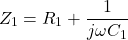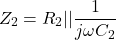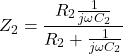(1)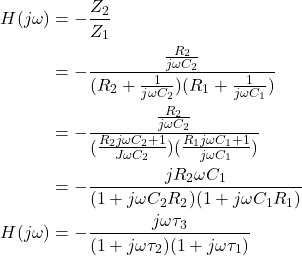Where,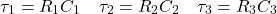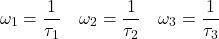For band pass filter, following condition must satisfy,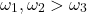## Band Pass Filter Cutoff Frequency

The band pass filter is a combination of two filters. Therefore, it has two cutoff frequencies. One cutoff frequency is derived from the high pass filter and it is denoted as Fc-high. The filter allows the signal which has the frequencies more than Fc-high. The value of Fc-high is calculated from the below formula.The second cutoff frequency is derived from the low pass filter and it is denoted as Fc-low. The filter allows the signal which has frequencies lower than the Fc-low. The value of Fc-low is calculated from the below formula.The filter operates between frequencies Fc-high and Fc-low. The range between these frequencies is known as bandwidth. Therefore, the bandwidth is defined as the below equation.The cutoff frequency of a high pass filter will define the lower value of bandwidth and the cutoff frequency of low pass filter will define the higher value of bandwidth.

## Band Pass Filter Bode Plot or Frequency Response

The above figure shows the bode plot or the frequency response and phase plot of band pass filter. The filter will allow the signal which has a frequency in between the bandwidth.

The filter will attenuate the signals which have frequency lower than the cutoff frequency of high pass filter. And till the signal reaches to FL, the output is increasing at the rate of +20 DB/Decade the same as the high pass filter.

After that, the output continuous at maximum gain until it reaches the cutoff frequency of low pass filter or at the point FH. Then the output will decrease at the rate of -20 DB/Decade the same as the low pass filter.

The band pass filter is a second-order filter because it has two reactive components in the circuit diagram. Therefore, the phase difference is twice the first-order filter and it is 180˚.

Until the center frequency, the output signal leads the input by 90˚. At the center frequency, the output signal is in phase with the input. Hence, the phase difference is 0˚.

After the center frequency, the output signal lags the input by 90˚.

## Ideal Band Pass Filter

An ideal band pass filter allows signal with exactly from FL similar to the step response. The signal allowing exactly at FL with the slope of 0 DB/Decade. And it abruptly attenuates the signals which have frequency more than FH.

The frequency response of the ideal band pass filter is as shown in the below figure. This type of response cannot result in an actual band pass filter.

### Band Pass Filter Equation

When the signal frequency is in the range of bandwidth, the filter will allow the signal with input impedance. And the output is zero when the signal frequency is outside of the bandwidth.

For band pass filter;

(2)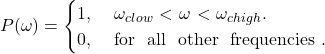## Band Pass Filter Applications

The application of band pass filter is as follows,

• Band pass filters are widely used in audio amplifier circuits. For example, the speaker is used to play only a desired range of frequencies and ignore the rest of the frequencies.
• It is used optics like LASER, LIDARS, etc.
• These filters are used in a communication system for choosing the signals with a particular bandwidth.
• It is used in audio signal processing.
• It is also used to optimize the signal to noise ratio and sensitivity of the receiver.

## Band Pass Filter Design Example

Now you are familiar with the band pass filter. Let’s design a filter for specific bandwidth. We will make a filter which allows the signals which have frequencies in the range of 80 Hz to 800 Hz.

F1 = 80 Hz
F2 = 800 Hz

For this example, we will make a simple passive RC filter for a given range of the frequency. So, we have to calculate the value of R1, C1, R2, and C2.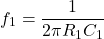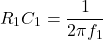Similarly,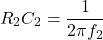We have to assume the value of resistance or capacitance. Here, we will assume the value of C1 and C2. For simple calculation, we will assume the same value for C1 and C2 and that is 10-6 F. And calculate the value of resistance according to this value of C1, C2, and F1, F2.Therefore,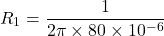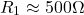Similarly,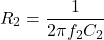Now, we have all values and by these values we can make a filter which allows the signals with specific bandwidth.

Want To Learn Faster? 🎓
Get electrical articles delivered to your inbox every week.
No credit card required—it’s 100% free.